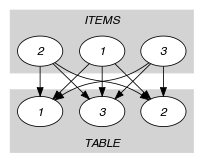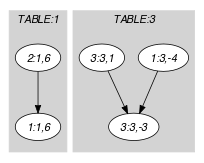## 5.192. indexed_sum

Origin

N. Beldiceanu

Constraint

$\mathrm{𝚒𝚗𝚍𝚎𝚡𝚎𝚍}_\mathrm{𝚜𝚞𝚖}\left(\mathrm{𝙸𝚃𝙴𝙼𝚂},\mathrm{𝚃𝙰𝙱𝙻𝙴}\right)$

Arguments
 $\mathrm{𝙸𝚃𝙴𝙼𝚂}$ $\mathrm{𝚌𝚘𝚕𝚕𝚎𝚌𝚝𝚒𝚘𝚗}\left(\mathrm{𝚒𝚗𝚍𝚎𝚡}-\mathrm{𝚍𝚟𝚊𝚛},\mathrm{𝚠𝚎𝚒𝚐𝚑𝚝}-\mathrm{𝚍𝚟𝚊𝚛}\right)$ $\mathrm{𝚃𝙰𝙱𝙻𝙴}$ $\mathrm{𝚌𝚘𝚕𝚕𝚎𝚌𝚝𝚒𝚘𝚗}\left(\mathrm{𝚒𝚗𝚍𝚎𝚡}-\mathrm{𝚒𝚗𝚝},\mathrm{𝚜𝚞𝚖𝚖𝚊𝚝𝚒𝚘𝚗}-\mathrm{𝚍𝚟𝚊𝚛}\right)$
Restrictions
 $|\mathrm{𝙸𝚃𝙴𝙼𝚂}|>0$ $|\mathrm{𝚃𝙰𝙱𝙻𝙴}|>0$ $\mathrm{𝚛𝚎𝚚𝚞𝚒𝚛𝚎𝚍}$$\left(\mathrm{𝙸𝚃𝙴𝙼𝚂},\left[\mathrm{𝚒𝚗𝚍𝚎𝚡},\mathrm{𝚠𝚎𝚒𝚐𝚑𝚝}\right]\right)$ $\mathrm{𝙸𝚃𝙴𝙼𝚂}.\mathrm{𝚒𝚗𝚍𝚎𝚡}\ge 1$ $\mathrm{𝙸𝚃𝙴𝙼𝚂}.\mathrm{𝚒𝚗𝚍𝚎𝚡}\le |\mathrm{𝚃𝙰𝙱𝙻𝙴}|$ $\mathrm{𝚛𝚎𝚚𝚞𝚒𝚛𝚎𝚍}$$\left(\mathrm{𝚃𝙰𝙱𝙻𝙴},\left[\mathrm{𝚒𝚗𝚍𝚎𝚡},\mathrm{𝚜𝚞𝚖𝚖𝚊𝚝𝚒𝚘𝚗}\right]\right)$ $\mathrm{𝚃𝙰𝙱𝙻𝙴}.\mathrm{𝚒𝚗𝚍𝚎𝚡}\ge 1$ $\mathrm{𝚃𝙰𝙱𝙻𝙴}.\mathrm{𝚒𝚗𝚍𝚎𝚡}\le |\mathrm{𝚃𝙰𝙱𝙻𝙴}|$ $\mathrm{𝚒𝚗𝚌𝚛𝚎𝚊𝚜𝚒𝚗𝚐}_\mathrm{𝚜𝚎𝚚}$$\left(\mathrm{𝚃𝙰𝙱𝙻𝙴},\mathrm{𝚒𝚗𝚍𝚎𝚡}\right)$
Purpose

Given several items of the collection $\mathrm{𝙸𝚃𝙴𝙼𝚂}$ (each of them having a specific fixed $\mathrm{𝚒𝚗𝚍𝚎𝚡}$ as well as a $\mathrm{𝚠𝚎𝚒𝚐𝚑𝚝}$ that may be negative or positive), and a table $\mathrm{𝚃𝙰𝙱𝙻𝙴}$ (each entry of $\mathrm{𝚃𝙰𝙱𝙻𝙴}$ corresponding to a $\mathrm{𝚜𝚞𝚖𝚖𝚊𝚝𝚒𝚘𝚗}$ variable), assign each item to an entry of $\mathrm{𝚃𝙰𝙱𝙻𝙴}$ so that the sum of the weights of the items assigned to that entry is equal to the corresponding $\mathrm{𝚜𝚞𝚖𝚖𝚊𝚝𝚒𝚘𝚗}$ variable.

Example
$\left(\begin{array}{c}〈\begin{array}{cc}\mathrm{𝚒𝚗𝚍𝚎𝚡}-3\hfill & \mathrm{𝚠𝚎𝚒𝚐𝚑𝚝}--4,\hfill \\ \mathrm{𝚒𝚗𝚍𝚎𝚡}-1\hfill & \mathrm{𝚠𝚎𝚒𝚐𝚑𝚝}-6,\hfill \\ \mathrm{𝚒𝚗𝚍𝚎𝚡}-3\hfill & \mathrm{𝚠𝚎𝚒𝚐𝚑𝚝}-1\hfill \end{array}〉,\hfill \\ 〈\begin{array}{cc}\mathrm{𝚒𝚗𝚍𝚎𝚡}-1\hfill & \mathrm{𝚜𝚞𝚖𝚖𝚊𝚝𝚒𝚘𝚗}-6,\hfill \\ \mathrm{𝚒𝚗𝚍𝚎𝚡}-2\hfill & \mathrm{𝚜𝚞𝚖𝚖𝚊𝚝𝚒𝚘𝚗}-0,\hfill \\ \mathrm{𝚒𝚗𝚍𝚎𝚡}-3\hfill & \mathrm{𝚜𝚞𝚖𝚖𝚊𝚝𝚒𝚘𝚗}--3\hfill \end{array}〉\hfill \end{array}\right)$

The $\mathrm{𝚒𝚗𝚍𝚎𝚡𝚎𝚍}_\mathrm{𝚜𝚞𝚖}$ constraint holds since the summation variables associated with each entry of $\mathrm{𝚃𝙰𝙱𝙻𝙴}$ are equal to the sum of the weights of the items assigned to the corresponding entry:

• $\mathrm{𝚃𝙰𝙱𝙻𝙴}\left[1\right].\mathrm{𝚜𝚞𝚖𝚖𝚊𝚝𝚒𝚘𝚗}=\mathrm{𝙸𝚃𝙴𝙼𝚂}\left[2\right].\mathrm{𝚠𝚎𝚒𝚐𝚑𝚝}=6$ (since $\mathrm{𝚃𝙰𝙱𝙻𝙴}\left[1\right].\mathrm{𝚒𝚗𝚍𝚎𝚡}=\mathrm{𝙸𝚃𝙴𝙼𝚂}\left[2\right].\mathrm{𝚒𝚗𝚍𝚎𝚡}=1$),

• $\mathrm{𝚃𝙰𝙱𝙻𝙴}\left[2\right].\mathrm{𝚜𝚞𝚖𝚖𝚊𝚝𝚒𝚘𝚗}=0$ (since $\mathrm{𝚃𝙰𝙱𝙻𝙴}\left[2\right].\mathrm{𝚒𝚗𝚍𝚎𝚡}=2$ does not occur as a value of the $\mathrm{𝚒𝚗𝚍𝚎𝚡}$ attribute of an item of $\mathrm{𝙸𝚃𝙴𝙼𝚂}$),

• $\mathrm{𝚃𝙰𝙱𝙻𝙴}\left[3\right].\mathrm{𝚜𝚞𝚖𝚖𝚊𝚝𝚒𝚘𝚗}=\mathrm{𝙸𝚃𝙴𝙼𝚂}\left[1\right].\mathrm{𝚠𝚎𝚒𝚐𝚑𝚝}+\mathrm{𝙸𝚃𝙴𝙼𝚂}\left[3\right].\mathrm{𝚠𝚎𝚒𝚐𝚑𝚝}=-4+1=-3$ (since $\mathrm{𝚃𝙰𝙱𝙻𝙴}\left[3\right].\mathrm{𝚒𝚗𝚍𝚎𝚡}=\mathrm{𝙸𝚃𝙴𝙼𝚂}\left[1\right].\mathrm{𝚒𝚗𝚍𝚎𝚡}=\mathrm{𝙸𝚃𝙴𝙼𝚂}\left[3\right].\mathrm{𝚒𝚗𝚍𝚎𝚡}=3$).

Typical
 $|\mathrm{𝙸𝚃𝙴𝙼𝚂}|>1$ $\mathrm{𝚛𝚊𝚗𝚐𝚎}$$\left(\mathrm{𝙸𝚃𝙴𝙼𝚂}.\mathrm{𝚒𝚗𝚍𝚎𝚡}\right)>1$ $|\mathrm{𝚃𝙰𝙱𝙻𝙴}|>1$ $\mathrm{𝚛𝚊𝚗𝚐𝚎}$$\left(\mathrm{𝚃𝙰𝙱𝙻𝙴}.\mathrm{𝚜𝚞𝚖𝚖𝚊𝚝𝚒𝚘𝚗}\right)>1$
Symmetries
• Items of $\mathrm{𝙸𝚃𝙴𝙼𝚂}$ are permutable.

• Items of $\mathrm{𝚃𝙰𝙱𝙻𝙴}$ are permutable.

Reformulation

The $\mathrm{𝚒𝚗𝚍𝚎𝚡𝚎𝚍}_\mathrm{𝚜𝚞𝚖}$$\left(\mathrm{𝙸𝚃𝙴𝙼𝚂},\mathrm{𝚃𝙰𝙱𝙻𝙴}\right)$ constraint can be expressed in term of a set of reified constraints and of $|\mathrm{𝚃𝙰𝙱𝙻𝙴}|$ arithmetic constraints (i.e., $\mathrm{𝚜𝚌𝚊𝚕𝚊𝚛}_\mathrm{𝚙𝚛𝚘𝚍𝚞𝚌𝚝}$ constraints).

1. For each item $\mathrm{𝙸𝚃𝙴𝙼𝚂}\left[i\right]$ $\left(i\in \left[1,|\mathrm{𝙸𝚃𝙴𝙼𝚂}|\right]\right)$ and for each table entry $j$ $\left(j\in \left[1,|\mathrm{𝚃𝙰𝙱𝙻𝙴}|\right]\right)$ of $\mathrm{𝚃𝙰𝙱𝙻𝙴}$ we create a 0-1 variable ${B}_{ij}$ that will be set to 1 if and only if $\mathrm{𝙸𝚃𝙴𝙼𝚂}\left[i\right].\mathrm{𝚒𝚗𝚍𝚎𝚡}$ is fixed to $j$ (i.e., ${B}_{ij}⇔\mathrm{𝙸𝚃𝙴𝙼𝚂}\left[i\right].\mathrm{𝚒𝚗𝚍𝚎𝚡}=j$).

2. For each entry $j$ of the table $\mathrm{𝚃𝙰𝙱𝙻𝙴}$, we impose the sum $\mathrm{𝙸𝚃𝙴𝙼𝚂}\left[1\right].\mathrm{𝚠𝚎𝚒𝚐𝚑𝚝}·{B}_{1j}+\mathrm{𝙸𝚃𝙴𝙼𝚂}\left[2\right].\mathrm{𝚠𝚎𝚒𝚐𝚑𝚝}·{B}_{2j}+\cdots +\mathrm{𝙸𝚃𝙴𝙼𝚂}\left[|\mathrm{𝙸𝚃𝙴𝙼𝚂}|\right].\mathrm{𝚠𝚎𝚒𝚐𝚑𝚝}·{B}_{|\mathrm{𝙸𝚃𝙴𝙼𝚂}|j}$ to be equal to $\mathrm{𝚃𝙰𝙱𝙻𝙴}\left[j\right].\mathrm{𝚜𝚞𝚖𝚖𝚊𝚝𝚒𝚘𝚗}$.

specialisation: $\mathrm{𝚋𝚒𝚗}_\mathrm{𝚙𝚊𝚌𝚔𝚒𝚗𝚐}$ (negative contribution not allowed, effective use variable for each bin replaced by an overall fixed capacity), $\mathrm{𝚋𝚒𝚗}_\mathrm{𝚙𝚊𝚌𝚔𝚒𝚗𝚐}_\mathrm{𝚌𝚊𝚙𝚊}$ (negative contribution not allowed, effective use variable for each bin replaced by a fixed capacity for each bin).

Keywords

For all items of $\mathrm{𝚃𝙰𝙱𝙻𝙴}$:

Arc input(s)

$\mathrm{𝙸𝚃𝙴𝙼𝚂}$ $\mathrm{𝚃𝙰𝙱𝙻𝙴}$

Arc generator
$\mathrm{𝑃𝑅𝑂𝐷𝑈𝐶𝑇}$$↦\mathrm{𝚌𝚘𝚕𝚕𝚎𝚌𝚝𝚒𝚘𝚗}\left(\mathrm{𝚒𝚝𝚎𝚖𝚜},\mathrm{𝚝𝚊𝚋𝚕𝚎}\right)$

Arc arity
Arc constraint(s)
$\mathrm{𝚒𝚝𝚎𝚖𝚜}.\mathrm{𝚒𝚗𝚍𝚎𝚡}=\mathrm{𝚝𝚊𝚋𝚕𝚎}.\mathrm{𝚒𝚗𝚍𝚎𝚡}$
Sets
$\begin{array}{c}\mathrm{𝖲𝖴𝖢𝖢}↦\hfill \\ \left[\begin{array}{c}\mathrm{𝚜𝚘𝚞𝚛𝚌𝚎},\hfill \\ \mathrm{𝚟𝚊𝚛𝚒𝚊𝚋𝚕𝚎𝚜}-\mathrm{𝚌𝚘𝚕}\left(\begin{array}{c}\mathrm{𝚅𝙰𝚁𝙸𝙰𝙱𝙻𝙴𝚂}-\mathrm{𝚌𝚘𝚕𝚕𝚎𝚌𝚝𝚒𝚘𝚗}\left(\mathrm{𝚟𝚊𝚛}-\mathrm{𝚍𝚟𝚊𝚛}\right),\hfill \\ \mathrm{𝚒𝚝𝚎𝚖}\left(\mathrm{𝚟𝚊𝚛}-\mathrm{𝙸𝚃𝙴𝙼𝚂}.\mathrm{𝚠𝚎𝚒𝚐𝚑𝚝}\right)\right]\hfill \end{array}\right)\hfill \end{array}\right]\hfill \end{array}$

Constraint(s) on sets
$\mathrm{𝚜𝚞𝚖}_\mathrm{𝚌𝚝𝚛}$$\left(\mathrm{𝚟𝚊𝚛𝚒𝚊𝚋𝚕𝚎𝚜},=,\mathrm{𝚃𝙰𝙱𝙻𝙴}.\mathrm{𝚜𝚞𝚖𝚖𝚊𝚝𝚒𝚘𝚗}\right)$
Graph model

We enforce the $\mathrm{𝚜𝚞𝚖}_\mathrm{𝚌𝚝𝚛}$ constraint on the weight of the items that are assigned to the same entry. Within the context of the Example slot, part (A) of Figure 5.192.1 shows the initial graphs associated with entries 1, 2 and 3 (i.e., one initial graph for each item of the $\mathrm{𝚃𝙰𝙱𝙻𝙴}$ collection). Part (B) of Figure 5.192.1 shows the corresponding final graphs associated with entries 1 and 3. Each source vertex of the final graph can be interpreted as an item assigned to a specific entry of $\mathrm{𝚃𝙰𝙱𝙻𝙴}$.

##### Figure 5.192.1. Initial and final graph of the $\mathrm{𝚒𝚗𝚍𝚎𝚡𝚎𝚍}_\mathrm{𝚜𝚞𝚖}$ constraint(a) (b)